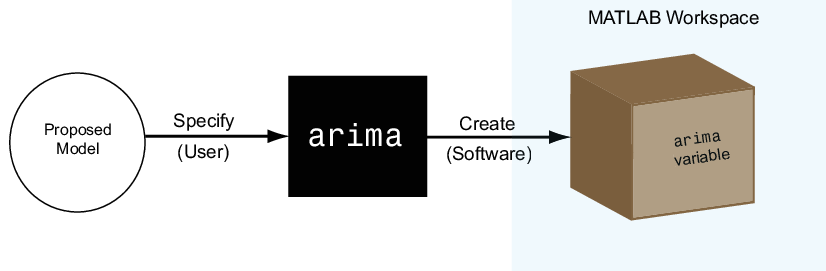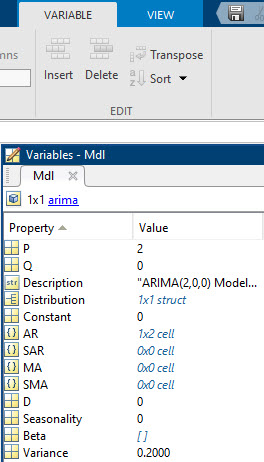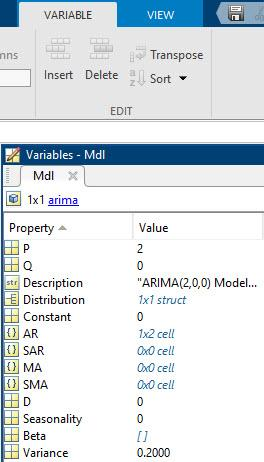## Econometrics Toolbox Model Objects, Properties, and Object Functions

### Model Objects

After you have a potential model for your data, you must specify the model to MATLAB® to proceed with your analysis. Econometrics Toolbox™ has model objects for storing discrete-time econometric models.

For univariate series, the available model objects are:

For multivariate series, the available model objects are:

• `varm` — for vector autoregression models optionally containing exogenous predictor variables

• `vecm` — for vector error-correction (cointegrated VARM) models optionally containing exogenous predictor variables

Econometrics Toolbox supports univariate Bayesian linear regression analysis. Bayesian linear regression model objects specify the joint prior distribution of the regression coefficients and disturbance variance. The available prior model objects are:

• `conjugateblm` — for the normal-inverse-gamma conjugate prior model. The regression coefficients and disturbance variance are dependent random variables.

• `semiconjugateblm` — for the normal-inverse-gamma semiconjugate prior model. The regression coefficients and disturbance variance are independent random variables.

• `diffuseblm` — the joint prior distribution is proportional to the inverse of the disturbance variance.

• `empiricalblm` — the joint prior distribution is specified by a random sample from the joint posterior distribution.

• `customblm` — the joint prior distribution is specified in a custom function that you declare.

To perform Bayesian variable selection, available prior model objects are:

• `mixconjugateblm` — for performing stochastic search variable selection (SSVS). The regression coefficients and disturbance variance are dependent random variables (the prior and posterior distributions are conjugate).

• `mixsemiconjugateblm` — for performing SSVS. The regression coefficients and disturbance variance are independent random variables (the prior and posterior distributions are semiconjugate).

• `lassoblm` — for performing Bayesian lasso regression.

Econometrics Toolbox supports modelling and analyzing discrete or continuous state Markov models. Available model objects are:

• `dtmc` — for discrete-time Markov chain models characterized by transition matrices.

• `ssm` — for continuous, multivariate state-space models optionally containing exogenous predictor variables

• `dssm` — for continuous, multivariate state-space models with diffuse initial states optionally containing exogenous predictor variables

To create a model object, specify the form of your model to one of the model functions (e.g., `arima` or `garch`). The function creates the model object of the corresponding type in the MATLAB workspace, as shown in the figure.You can work with model objects as you would with any other variable in MATLAB. For example, you can assign the object variable a name, view it in the MATLAB Workspace, and display its value in the Command Window by typing its name.

This image shows a workspace containing an `arima` model named `Mdl`.### Model Properties

A model object holds all the information necessary to estimate, simulate, and forecast econometric models. This information includes the:

• Parametric form of the model

• Number of model parameters (e.g., the degree of the model)

• Innovation distribution (Gaussian or Student’s t)

• Amount of presample data needed to initialize the model

Such pieces of information are properties of the model, which are stored as fields within the model object. In this way, a model object resembles a MATLAB data structure (`struct` array).

The five model types—`arima`, `garch`, `egarch`, `gjr`, and `regARIMA`—have properties according to the econometric models they support. Each property has a predefined name, which you cannot change.

For example, `arima` supports conditional mean models (multiplicative and additive AR, MA, ARMA, ARIMA, and ARIMAX processes). Every `arima` model object has these properties, shown with their corresponding names.

Property NameProperty Description
`Constant`Model constant
`AR`Nonseasonal AR coefficients
`MA`Nonseasonal MA coefficients
`SAR`Seasonal AR coefficients (in a multiplicative model)
`SMA`Seasonal MA coefficients (in a multiplicative model)
`D`Degree of nonseasonal differencing
`Seasonality`Degree of seasonal differencing
`Variance`Variance of the innovation distribution
`Distribution`Parametric family of the innovation distribution
`P`Amount of presample data needed to initialize the AR component of the model
`Q`Amount of presample data needed to initialize the MA component of the model

When a model object exists in the workspace, double-click its name in the Workspace window to open the Variable Editor. The Variable Editor shows all model properties and their names.Notice that in addition to a name, each property has a value.

### Specify Models

Specify a model by assigning values to model properties. You do not need, nor are you able, to specify a value for every property. The constructor function assigns default values to any properties you do not, or cannot, specify.

Tip

It is good practice to be aware of the default property values for any model you create.

In addition to having a predefined name, each model property has a predefined data type. When assigning or modifying a property’s value, the assignment must be consistent with the property data type.

For example, the `arima` properties have these data types.

Property NameProperty Data Type
`Constant`Scalar
`AR`Cell array
`MA`Cell array
`SAR`Cell array
`SMA`Cell array
`D`Nonnegative integer
`Seasonality`Nonnegative integer
`Variance`Positive scalar
`Distribution``struct` array
`P`Nonnegative integer (you cannot specify)
`Q`Nonnegative integer (you cannot specify)

#### Specify an AR(2) Model

To illustrate assigning property values, consider specifying the AR(2) model

`${y}_{t}=0.8{y}_{t-1}-0.2{y}_{t-2}+{\epsilon }_{t},$`

where the innovations are independent and identically distributed normal random variables with mean 0 and variance 0.2. Because the equation is a conditional mean model, use `arima` to create an object that represents the model. Assign values to model properties by using name-value pair arguments.

This model has two AR coefficients, 0.8 and -0.2. Assign these values to the property `AR` as a cell array, `{0.8,-0.2}`. Assign the value `0.2` to `Variance`, and `0` to `Constant`. You do not need to assign a value to `Distribution` because the default innovation distribution is `'Gaussian'`. There are no MA terms, seasonal terms, or degrees of integration, so do not assign values to these properties. You cannot specify values for the properties `P` and `Q`.

In summary, specify the model as follows:

`Mdl = arima('AR',{0.8,-0.2},'Variance',0.2,'Constant',0)`
```Mdl = arima with properties: Description: "ARIMA(2,0,0) Model (Gaussian Distribution)" Distribution: Name = "Gaussian" P: 2 D: 0 Q: 0 Constant: 0 AR: {0.8 -0.2} at lags [1 2] SAR: {} MA: {} SMA: {} Seasonality: 0 Beta: [1×0] Variance: 0.2 ```

The output displays the value of the created model, `Mdl`. Notice that the property `Seasonality` is not in the output. `Seasonality` only displays for models with seasonal integration. The property is still present, however, as seen in the Variable Editor.`Mdl` has values for every `arima` property, even though the specification included only three. `arima` assigns default values for the unspecified properties. The values of `SAR`, `MA`, and `SMA` are empty cell arrays because the model has no seasonal or MA terms. The values of `D` and `Seasonality` are `0` because there is no nonseasonal or seasonal differencing. `arima` sets:

• `P` equal to `2`, the number of presample observations needed to initialize an AR(2) model.

• `Q` equal to `0` because there is no MA component to the model (i.e., no presample innovations are needed).

#### Specify a GARCH(1,1) Model

As another illustration, consider specifying the GARCH(1,1) model

`${y}_{t}={\epsilon }_{t},$`

where

`$\begin{array}{rcl}{\epsilon }_{t}& =& {\sigma }_{t}{z}_{t}\\ {\sigma }_{t}^{2}& =& \kappa +{\gamma }_{1}{\sigma }_{t-1}^{2}+{\alpha }_{1}{\epsilon }_{t-1}^{2}\end{array}$`

Assume ${z}_{t}$ follows a standard normal distribution.

This model has one GARCH coefficient (corresponding to the lagged variance term) and one ARCH coefficient (corresponding to the lagged squared innovation term), both with unknown values. To specify this model, enter:

`Mdl = garch('GARCH',NaN,'ARCH',NaN)`
```Mdl = garch with properties: Description: "GARCH(1,1) Conditional Variance Model (Gaussian Distribution)" Distribution: Name = "Gaussian" P: 1 Q: 1 Constant: NaN GARCH: {NaN} at lag  ARCH: {NaN} at lag  Offset: 0 ```

The default value for the constant term is also `NaN`. Parameters with `NaN` values need to be estimated or otherwise specified before you can forecast or simulate the model. There is also a shorthand syntax to create a default GARCH(1,1) model:

`Mdl = garch(1,1)`
```Mdl = garch with properties: Description: "GARCH(1,1) Conditional Variance Model (Gaussian Distribution)" Distribution: Name = "Gaussian" P: 1 Q: 1 Constant: NaN GARCH: {NaN} at lag  ARCH: {NaN} at lag  Offset: 0 ```

The shorthand syntax returns a GARCH model with one GARCH coefficient and one ARCH coefficient, with default `NaN` values.

### Retrieve Model Properties

The property values in an existing model are retrievable. Working with models resembles working with `struct` arrays because you can access model properties using dot notation. That is, type the model name, then the property name, separated by `'.'` (a period).

For example, consider the `arima` model with this AR(2) specification:

`Mdl = arima('AR',{0.8,-0.2},'Variance',0.2,'Constant',0);`

To display the value of the property `AR` for the created model, enter:

`arCoefficients = Mdl.AR`
```arCoefficients=1×2 cell array {[0.8000]} {[-0.2000]} ```

`AR` is a cell array, so you must use cell-array syntax. The coefficient cell arrays are lag-indexed, so entering

`secondARCoefficient = Mdl.AR{2}`
```secondARCoefficient = -0.2000 ```

returns the coefficient at lag 2. You can also assign any property value to a new variable:

`ar = Mdl.AR`
```ar=1×2 cell array {[0.8000]} {[-0.2000]} ```

### Modify Model Properties

You can also modify model properties using dot notation. For example, consider this AR(2) specification:

`Mdl = arima('AR',{0.8,-0.2},'Variance',0.2,'Constant',0)`
```Mdl = arima with properties: Description: "ARIMA(2,0,0) Model (Gaussian Distribution)" Distribution: Name = "Gaussian" P: 2 D: 0 Q: 0 Constant: 0 AR: {0.8 -0.2} at lags [1 2] SAR: {} MA: {} SMA: {} Seasonality: 0 Beta: [1×0] Variance: 0.2 ```

The created model has the default Gaussian innovation distribution. Change the innovation distribution to a Student's t distribution with eight degrees of freedom. The data type for `Distribution` is a `struct` array.

`Mdl.Distribution = struct('Name','t','DoF',8)`
```Mdl = arima with properties: Description: "ARIMA(2,0,0) Model (t Distribution)" Distribution: Name = "t", DoF = 8 P: 2 D: 0 Q: 0 Constant: 0 AR: {0.8 -0.2} at lags [1 2] SAR: {} MA: {} SMA: {} Seasonality: 0 Beta: [1×0] Variance: 0.2 ```

The variable `Mdl` is updated accordingly.

### Object Functions

Object functions are functions that accept model objects as inputs. In Econometrics Toolbox, these functions, which represent steps in an econometrics analysis workflow, accept any of the model objects included in the toolbox:

• `estimate`

• `forecast`

• `simulate`

Model that you can fit to data have these three methods in common, but the model objects in the toolbox can have other object functions.

Object functions can distinguish between model objects (e.g., an `arima` model vs. a `garch` model). That is, some object functions accept different optional inputs and return different outputs depending on the type of model that is input.

Find object function reference pages for a specific model by entering, for example, `doc arima/estimate`.